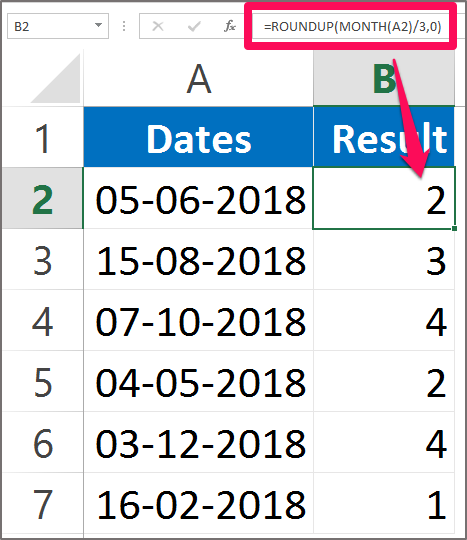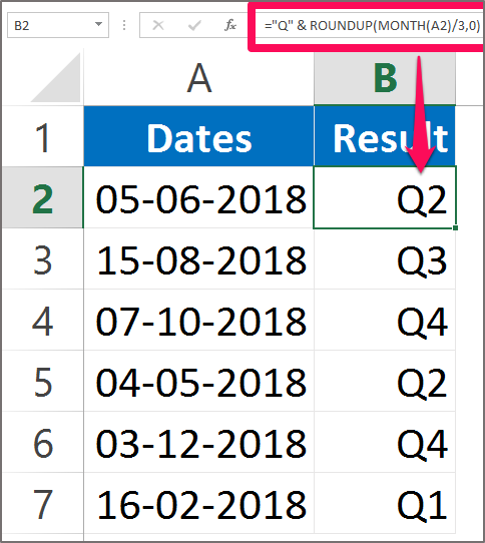# Quarter formula in Excel – Excel Formulas in Hindi

## Quarter Formula in Excel | Convert month to quarter

In Excel has various in-built functions that returns years, Month number, and name of the Month, Week number, Weekday or any other days. But there is no built-in function that can calculate and return the Quarter Number. Quarters are difficult to find because it depends on the year ending date. Quarter formula in Excel.

Usually Calendar year is taken into consideration while calculating the Quarters for the year. Calendar date ends on 31st December. A year is divided into four quarters. This tutorial will explain you how to find the quarter from the dates given.

You need to use Roundup function along with the Month function to calculate the Quarter.

The syntax for the Roundup function in Excel is:

ROUNDUP(number, num_digits)

The Roundup Function in this formula rounds a number to the nearest whole number. The Roundup function will always round number 1-9 upwards.

The syntax for the Month function in Excel is:

MONTH(serial_number)

The Month function in this formula returns the number of the month. You can enter the Month function by using quotation or by using cell reference.

Why is 3 in the formula? 3 months is in the formula because a quarter has 3 months and we have to divide the year by 3 to get quarter answer.